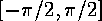<b>double atan(double x)</b>

Document: The C Standard Librarydouble atan2(double ydouble x)Mathematical Functions: math.hdouble acos(double x)

## double atan(double x)

The return value is the inverse tangent or arctangent of x. The return value will be expressed in radians and lie in the interval.

McMullin@eeng.dcu.ie
Fri Mar 29 14:35:38 GMT 1996